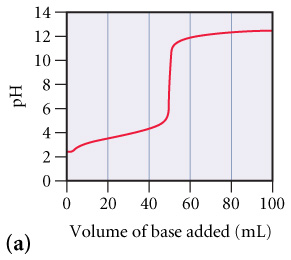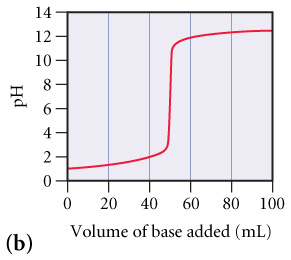# Problem: The graphs below labeled (a) and (b) show the titration curves for two equal-volume samples of monoprotic acids, one weak and one strong. Both titrations were carried out with the same concentration of strong base.What is the approximate pH at the equivalence point of (a) curve?What is the approximate pH at the equivalence point of (b) curve?Which curve corresponds to the titration of the strong acid and which one to the titration of the weak acid?

###### FREE Expert Solution

Weak acid and strong base titration

• pH gradually increases as it approaches the equivalence point
• pH at the equivalence point is more than 7.00

Strong acid and strong base titration

• pH rapidly increases as it approaches the equivalence point
• pH at the equivalence point is equal to 7.00

Part A.

The steepest point in the curve will be the equivalence point.

• This is basically the turning point in curve.
• This is usualy the middle point of the horizontal segment.
• Then we simply trace to the pH axis to identify the value.

81% (479 ratings)###### Problem Details

The graphs below labeled (a) and (b) show the titration curves for two equal-volume samples of monoprotic acids, one weak and one strong. Both titrations were carried out with the same concentration of strong base.

What is the approximate pH at the equivalence point of (a) curve?What is the approximate pH at the equivalence point of (b) curve?Which curve corresponds to the titration of the strong acid and which one to the titration of the weak acid?

Frequently Asked Questions

What scientific concept do you need to know in order to solve this problem?

Our tutors have indicated that to solve this problem you will need to apply the Weak Acid Strong Base Titrations concept. You can view video lessons to learn Weak Acid Strong Base Titrations. Or if you need more Weak Acid Strong Base Titrations practice, you can also practice Weak Acid Strong Base Titrations practice problems.